## Disclaimer

This information HAS errors and is made available WITHOUT ANY WARRANTY OF ANY KIND and without even the implied warranty of MERCHANTABILITY or FITNESS FOR A PARTICULAR PURPOSE. It is not permissible to be read by anyone who has ever met a lawyer or attorney. Use is confined to Engineers with more than 370 course hours of engineering.
If you see an error contact:
+1(785) 841 3089
inform@xtronics.com

# Electronic Formulas### Power

• W = Watt
• J = Joule
• S = Second

,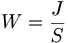### Transformer inductance and coupling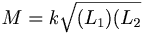Where:

• M = Mutual inductance in Henrys
• k = coefficient of coupling
• L_1 Inductance of first winding
• L_2 Inductance of second winding

#### Turns ratio

For Iron Core ,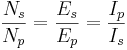Where:

• p subscript refers to Primary winding
• s subscript refers to secondary winding
• N = number of turns

#### Ohms law in magnetics

Where:

• F = repulsive force
• r = distance between poles
• μ permeability ( μ of air = 1)
• H = Magnetizing Force in Oersted
• mmf = Magnetic Motive Force - in Gilberts
• Orested = Gilberts/CM
• β = Flux density in Gauss
• φ = Total flux density in Maxwells
• r = Reluctance
• P = Permeance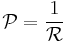mmf = φR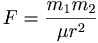,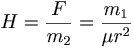### Impedance

Where

• XL refers to inductive reactance
• XC refers to capacitive reactance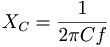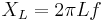#### Impedance through a transformer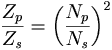#### Series RCL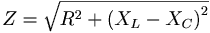#### Parallel RCL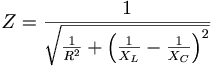### Parallel LC Resonance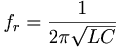#### Q or Quality factor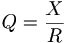### Time constance

Τ = time in seconds to 2/3 rise

Τ = RC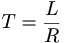### Power factor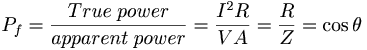#### Standing Wave Ratio (SWR)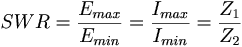### Modulation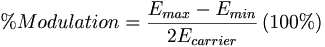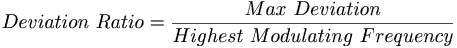Deviation = modulating index Deviation ratio = Deviation / highest modulating frequency

### Transistors

Ic = Ibβ

or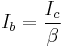• Where

β = beta [/itex] Ib = Base current Ic = Collector current

Transconductance
Transconductance is a contraction of transfer conductance. The old unit of conductance, the mho (ohm spelled backwards), was replaced by the SI unit, the siemens, with the symbol S (1 siemens = 1 ampere per volt).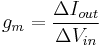For small signal alternating current, the Transconductance is estimated: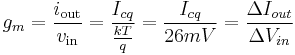Where :

• gm = small signal transconductance
•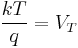Thermal voltage
• k Boltzmann constant ,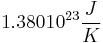• Icq = quiescent point, Q-point, or bias point

Distortion begins somewhere once the base voltage exceeds about 5 to 15mVp-p

If we look at large signals we must use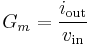Where :

Gm = Large signal Transconductance (not to be confused with gm
• Small-signal-input-resistance ( Rπ )for a common emitter amp with the emitter AC grounded: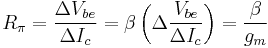Which means that input resistance goes up with β

• Large-signal-Input-resistance ( RΠ )for a common emitter amp with the emitter AC grounded: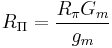### Ebers-Moll equation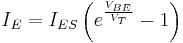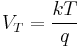(approximately 26 mV at 300 K ≈ room temperature).

where

• VT is the Boltzmann constant kT / q
• q Electronic Charge
• TTemperature in K
• IE is the emitter current
• IC is the collector current
• αF is the common base forward short circuit current gain (0.98 to 0.998)
• IES is the reverse saturation current of the base–emitter diode (on the order of 10−15 to 10−12 amperes)
• VBE is the base–emitter voltage

## ESR formulas

 Top Page wiki Index

Email